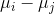# GRE Subject Test: Psychology : Statistical Procedures

## Example Questions

← Previous 1 3 4

### Example Question #1 : Statistical Procedures

Which of the following statistical tools has one mode and 99.7% of the data falls within three standard deviations of the mean?

Venn diagram

Learning curve

Chi-squared test

Scatter plots

Bell curve

Bell curve

Explanation:

A bell curve, or normal distribution, is a symmetrical curve with the mode (which also correlates to the mean and median) at the highest point of the curve. The mode is the value most frequently occuring in a set of variables. The mean is the average of a set of values. How spread out the curve is determined by the data set's standard deviation.

A learning curve plots one variable on the x-axis and another on the y-axis. An example of a learning curve could be experience plotted against learning. A person who learns quickly will have a learning curve convex to the vertical axis. A Venn diagram consists of two overlapping circles. In the overlap area, items that are similar to the data sets are included. Scatter plots show the observed relationships between different variables. A chi-squared test compares observed data with data the experimenter would expect to obtain.

### Example Question #1 : Statistical Procedures

A statistician who determines that the independent variable has no effect when it really does have an effect has committed which of the following errors?

Effect size error

Type I error

Type II error

A sampling error

Type II error

Explanation:

A type II error means that the experimenter has failed to recognize an effect that is present. On the other hand, a type I error indicates that the experimenter has mistakenly stated that the independent variable has an effect when it has no real effect.

### Example Question #2 : Statistical Procedures

Which of the following post hoc tests applies simultaneously to all pairwise comparisons, and is considered conservative if sample sizes are unequal?

Rodger's method

Newman-Keuls method

Tukey's range test

Fisher's least significant difference (LSD)

Scheffe's method

Tukey's range test

Explanation:

Named after John Tukey, the Tukey test is based on a studentized range distribution included with many standard psychological and statistical textbooks today. It is flexible enough to be used with all types of pairwise comparisons, including ANOVA and multiple comparison tests; however, it suffers when sample sizes are unequal.

### Example Question #1 : Statistical Procedures

Which of the following kinds of diagrams is best to display nominal data?

Frequency polygon

Histogram

Design

Bar chart

Bar chart

Explanation:

Nominal data is best represented by a bar chart. Nominal data can be variables such as religion. This is because there is no inherent rank. No one religion is statistically “better” than the other; therefore, it would be impossible to place one higher up than another in a rank order scheme.

### Example Question #2 : Statistical Procedures

Which of the following can be described as the variable that is changed in an experiment in order to determine its effects on other variables?

Experiment

Dependent variable

Correlation

Independent variable

Independent variable

Explanation:

The correct choice is “independent variable.” The independent variable is changed in order to observe the changes, if any, that it has on the dependent variable or variables. The dependent variable is defined as the variable that changes in response to the independent variable.

### Example Question #3 : Statistical Procedures

Which of the following is best defined as the distance that any point is from the mean on a normal distribution?

Standard deviation

Dependent variable

Z-score

Ratio

Standard deviation

Explanation:

The distance that any point is from the mean is known as the standard deviation. When looking at a normal distribution, the mean is the middle or arithmetic average of the set and the deviation measures the spread of the data points from this central tendency.

### Example Question #1 : Statistical Procedures

Which of the following is defined as the measurement of variables that have an inherent natural order?

Ratio

Interval

Nominal

Ordinal

Ordinal

Explanation:

Ordinal data is used when variables that have an inherent natural order, such as the collegiate class structure: freshman, sophomore, junior, senior.  One must complete every level in order to transcend to the next. Nominal data deals with variables such as religion that do not have an inherent natural order. Interval data deals with things like temperature. Last, ratio data would deal with things like time.

### Example Question #2 : Statistical Procedures

Which of the following could be defined as all of the members of a group under consideration in a study?

Sample

Parameter

Statistic

Population

Population

Explanation:

All of the members of a group under consideration are referred to as a population. A sample is the subset of the group under consideration. “Statistic” or “parameter” would be incorrect choices because a statistic represents a measure of a sample, while a parameter refers to a measure of a population.

### Example Question #1 : Statistical Procedures

Which of the following best describes a normal distribution?

Unimodal, asymptotic, and asymmetric

Bimodal, symptotic and asymmetric

Unimodal, asymptotic, and symmetric

Bimodal, asymptotic, and symmetric

Unimodal, asymptotic, and asymmetric

Explanation:

A normal distribution is asymptotic because it approaches a curve closely. It is unimodal because of its singular mode, and it is symmetric because both sides are mirror images of each other. There are other kinds of distributions like skewed distributions that are unimodal, asymptotic, and asymmetrical. These descriptions can vary depending on the shape of the distribution.

### Example Question #4 : Statistical Procedures

A parameter measures components of which of the following?

Statistic

Variable

Sample

Population